• beizer曲线
2019-05-22 17:35:44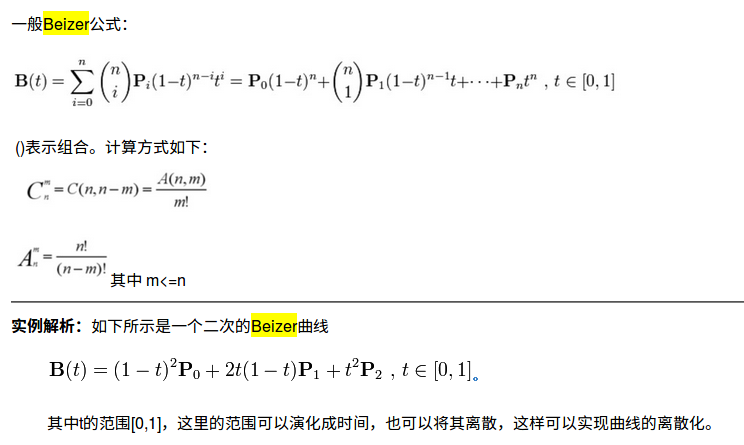已知曲线上的三个拟合曲线：

C++:


//Beizer曲线的三个点分别对应ptLeft(左侧点),ptMid(中间的某一点),ptRight(右侧点)

Point2f ptLeft,ptMid,ptRight;
//曲线中的t值，中间点在左右两点的
float locRatio = (ptMid.x-ptLeft.x)/(ptRight.x -ptLeft.x);
//求出控制点位置
Point2f ptControl;
ptControl.x =(ptMid.x -(1-locRatio)*(1-locRatio)*ptLeft.x-locRatio*locRatio*ptRight.x)/2/locRatio/(1-locRatio);
ptControl.y =(ptMid.y -(1-locRatio)*(1-locRatio)*ptLeft.y-locRatio*locRatio*ptRight.y)/2/locRatio/(1-locRatio);
//显示拟合出的曲线
Point2f calcuatePoint;
//以固定固定长度拉伸ptLeft,ptRight之间长度，改变曲线

for (float idx =1;idx <ptRight.x -ptLeft.x;idx++)
{
locRatio = idx/(ptRight.x-ptLeft.x-1);
//这里求出对应的每一处x，对应新的y值

calcuatePoint.x = ptLeft.x +idx;
calcuatePoint.y =(1-locRatio)*(1-locRatio)*ptLeft.y +2*locRatio*(1-locRatio)*ptControl.y +locRatio*locRatio*ptRight.y;
}


Python:



def beizerCurve(pt_left,pt_mid,pt_right):

locRatio = float(pt_mid - pt_left) / float(pt_right - pt_left);

#控制点位置
ptControl=[0,0]
ptControl = (pt_mid - (1 - locRatio) * (
1 - locRatio) * pt_left - locRatio * locRatio * pt_right) / 2 / locRatio / (1 - locRatio);
ptControl = (pt_mid - (1 - locRatio) * (
1 - locRatio) * pt_left - locRatio * locRatio * pt_right) / 2 / locRatio / (1 - locRatio);

curve =[]
for idx in range(pt_right - pt_left):
calcuatePoint = [0, 0]
locRatio = float(idx) / float(pt_right - pt_left-1)
calcuatePoint = pt_left +idx
calcuatePoint = int((1.0-locRatio)**2*pt_left+2*locRatio*(1-locRatio)*ptControl+locRatio**2*pt_right)
curve.append(calcuatePoint)

return curve

更多相关内容
• 内容：设计一曲面模型 目的： （1）掌握三次 Beizer 曲线的参数多项式的表示和 De Casteljau 递推算法； （2）掌握 Beizer 曲面的参数多项式表示以及生成曲面方法； 要求： （1）使用Beizer 自由曲面生成技术设计一...
• beizer算法的vb版 贝塞尔曲线(Bézier curve)，又称贝兹曲线或贝济埃曲线，是应用于二维图形应用程序的数学曲线。一般的矢量图形软件通过它来精确画出曲线，贝兹曲线由线段与节点组成，节点是可拖动的支点，线段像可...
• 绘制Beizer曲线用的。 程序里面的LONG型请大家改为DOUBLE类型，否则最多只能绘制13次的曲线。 使用的是最原始的算法，压缩包里的WORD文档有说明。 如果使用De Casteljau算法效果要好得多。
• 三次Beizer曲线方程介绍Beizer曲线的一些特性这里不再赘述，大家可以去网上查看一些资料，很详细。最近用到轮廓拟合，所以用三次Beizer曲线效果还可以，有插值和近似拟合（插值就是曲线过点，近似拟合则不过点），就...

## 1 三次Beizer曲线方程介绍

Beizer曲线的一些特性这里不再赘述，大家可以去网上查看一些资料，很详细。最近用到轮廓拟合，所以用三次Beizer曲线效果还可以，有插值和近似拟合（插值就是曲线过点，近似拟合则不过点），就学习了一下。我是做的Beizer曲线插值，插值和近视拟合无非就是控制点选取不一样。
Beizer总方程为
PiKni(t) $\sum P_{i}K_{i}^{n}(t)$ (1)，
三次Beizer曲线方程：
Bn(t)=P0(1t)3+3P1t(1t)2+3P2t2(1t)+P3t3,tϵ[0,1] $B_{n}(t)=P_{0}(1-t)^{3}+3P_{1}t(1-t)^{2}+3P_{2}t^{2}(1-t)+P_{3}t^{3},t\epsilon [0,1]$ (2)，
这里的 P1 $P_1$ P2 $P_2$就是所谓的控制点A、B点。关于控制点AB的求法很多种，我是采用参数设定法构造控制点，思路我是参考百度文库一篇文章，“确定控制点文章链接”。文章写的很详细，对于闭合轮廓的话就想象成一个循环，第0个点的前两个点为n-1和n-2，第n-1个点的后两个点为0和1，文章很好理解。对于参数a和b，通常都是0.25，但我看别人论文中有a=0.125，b=0.05，拟合出来的效果很接近真实曲线，所以我也是采用这个参数。

## 2 代码实现

上述讲了三次Beizer曲线方程，用在轮廓拟合中怎么实现呢。当然，你先得找到轮廓中的特征点，然后根据两个相邻的特征点拟合成一段三次Beizer曲线，控制点AB是借助周围几个点得到的。思路大概就是这样的。下面讲一下具体步骤。
###2.1 得到控制点AB
控制点AB方程在刚才那篇百度文科文章中有，所以直接根据那个方程来编写代码，下面是我求控制点AB的代码，很简单，大家有需要的可以参考。

//求得控制点AB
void ControlAB(double *Xi,double *Yi, double *Ai_x,double *Ai_y, double *Bi_x,double *Bi_y,int n, double a, double b,int boundType)
{
if(boundType==1)
{
Ai_x=Xi+(Xi-Xi[n-1])*a;
Ai_y=Yi+(Yi-Yi[n-1])*a;

Bi_x[n-2]=Xi[n-1]-(Xi-Xi[n-2])*b;
Bi_y[n-2]=Yi[n-1]-(Yi-Yi[n-2])*b;

Ai_x[n-1]=Xi[n-1]+(Xi-Xi[n-2])*a;
Ai_y[n-1]=Yi[n-1]+(Yi-Yi[n-2])*a;
Bi_x[n-1]=Xi-(Xi-Xi[n-1])*b;
Bi_y[n-1]=Yi-(Yi-Yi[n-1])*b;
}
for(int i=1;i<n-1;i++)
{
Ai_x[i]=Xi[i]+(Xi[i+1]-Xi[i-1])*a;
Ai_y[i]=Yi[i]+(Yi[i+1]-Yi[i-1])*a;
}
for(int i=0;i<n-2;i++)
{
Bi_x[i]=Xi[i+1]-(Xi[i+2]-Xi[i])*b;
Bi_y[i]=Yi[i+1]-(Yi[i+2]-Yi[i])*b;
}
}

代码注意：代码不要瞎贴，这里有些自定义数组，Xi、Yi是点的x、y。

###2.2 拟合曲线生成与绘制
求得AB控制点，可以将 P1 $P_1$ P2 $P_2$、A和B代入方程(2)中，得到 P1 $P_1$ P2 $P_2$中间的一段三次Beizer曲线方程，就拟合出来了。这时需要看到效果。我用的是C++的MFC画出来的曲线，当然也可以Python，Python一搜有很多例子，网上可以荡到的。我画二维曲线用的是MFC，大家可以参考我的博客“MFC绘制二维曲线”，给个我实验拟合出来的闭合轮廓，其中参数a=0.125，b=0.05。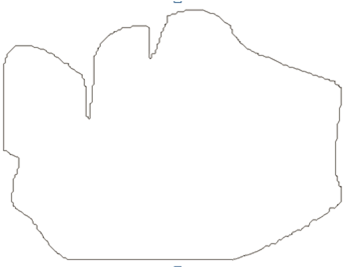原轮廓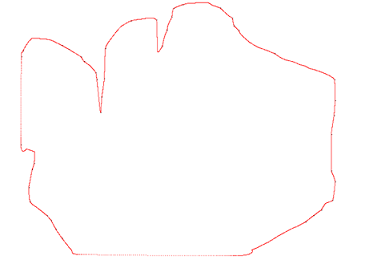拟合轮廓

可以看到，拟合效果还是不错的。图中的小黑点是我给的特征点，MFC画出来就是这个样子。

## 3 总结

三次Beizer曲线拟合算法还是很简单的，主要是控制点的选取，还有最关键的特征点选取。特征点是最基本的。当然Beizer也有一些不足之处，对于非闭合轮廓的情况拟合情况不是很理想，这就需要其他的曲线拟合，我会再发一篇B样条曲线拟合算法的。

展开全文openCV 轮廓拟合
• 使用C语言编写，borland库 自己的作品，为了积分才上传的。。。
• 关键代码： Bezier曲线： void CalcBZPoints(){ float a0,a1,a2,a3,b0,b1,b2,b3; a0=pt.x; a1=-3*pt.x+3*pt.x; a2=3*pt.x-6*pt.x+3*pt.x; a3=-pt.x+3*pt.x-3*...
关键代码：
Bezier曲线：
void CalcBZPoints(){
float a0,a1,a2,a3,b0,b1,b2,b3;
a0=pt.x;
a1=-3*pt.x+3*pt.x;
a2=3*pt.x-6*pt.x+3*pt.x;
a3=-pt.x+3*pt.x-3*pt.x+pt.x;
b0=pt.y;
b1=-3*pt.y+3*pt.y;
b2=3*pt.y-6*pt.y+3*pt.y;
b3=-pt.y+3*pt.y-3*pt.y+pt.y;
float t = 0;
float dt = 0.01;
for(int i = 0; t<1.1; t+=0.1, i++){
bz[i].x = a0+a1*t+a2*t*t+a3*t*t*t;
bz[i].y = b0+b1*t+b2*t*t+b3*t*t*t;
}
}
画点函数：
void ControlPoint(vector<Point> vpt){
glPointSize(2);//点的粗细
for(int i=0; i<vpt.size(); i++){
glBegin (GL_POINTS);
glColor3f (1.0f, 1.0f, 0.5f);//控制点颜色
glVertex2i (vpt[i].x,vpt[i].y);
glEnd ();
}
}
连线函数：
void PolylineGL(Point *pt, int num) {
glBegin (GL_LINE_STRIP);
for(int i=0;i<num;i++){
glColor3f (0.0f, 0.0f, 0.0f);//线的颜色
glVertex2i (pt[i].x,pt[i].y);
}
glEnd ();
}
画点画线：
void myDisplay(){
glColor3f (0.0f, 0.0f, 0.0f);//线的颜色为黑色
glPointSize(5);//点的宽度
if (vpt.size() > 0) {
ControlPoint(vpt);
}
if(bDraw){
CalcBZPoints();
PolylineGL(bz, 11);//画曲线
}
glFlush();
}
初始：
void Init(){
glClearColor(1.0, 1.0, 1.0, 0.0);//背景颜色
glClear(GL_COLOR_BUFFER_BIT);//清除当前帧的颜色-黑色
printf("Please Click left button of mouse to input control point of Bezier Curve!\n");
}
鼠标控制四个控制点：
void mouse(int button, int state, int x, int y){
switch (button){
case GLUT_LEFT_BUTTON:
if (state == GLUT_DOWN){
if (nInput == 0){
pt.x = x;
pt.y = 480 - y;
printf("(%.3f,%.3f)",pt.x/640.0,pt.y/480.0);//输出控制顶点坐标0
nInput = 1;
vpt.clear();
vpt.push_back(pt);
bDraw = false;
glutPostRedisplay();
}
else if (nInput == 1){
pt.x = x;
pt.y = 480 - y;
printf("(%.3f,%.3f)",pt.x/640.0,pt.y/480.0);//输出控制顶点坐标1
vpt.push_back(pt);
nInput = 2;
glutPostRedisplay();//
}
else if (nInput == 2){
pt.x = x;
pt.y = 480 - y;
printf("(%.3f,%.3f)",pt.x/640.0,pt.y/480.0);//输出控制顶点坐标2
vpt.push_back(pt);
nInput = 3;
glutPostRedisplay();//
}
else if (nInput == 3){
pt.x = x;
pt.y = 480 - y;
printf("(%.3f,%.3f)\n",pt.x/640.0,pt.y/480.0);//输出控制顶点坐标3
bDraw = true;
vpt.push_back(pt);
nInput = 0;
glutPostRedisplay();
}
}
break;
default:
break;
}
}
void KeyboardFunc(unsigned char Key,int x,int y){
if(Key == 'w' || Key == 'W'){ //按键清屏
glClear(GL_COLOR_BUFFER_BIT);//清屏
printf("清屏一次\n");
}
if(Key == 'x' || Key == 'X'){ //按键清除上次所画区域——将所画曲线变为背景色
glColor3f (1.0f, 1.0f, 1.0f);
if (vpt.size() > 0) {
ControlPoint(vpt);
}
if(bDraw){
CalcBZPoints();
glBegin (GL_LINE_STRIP);
for(int i=0;i<101;i++){
glColor3f (1.0f, 1.0f, 1.0f);//线颜色
glVertex2i (bz[i].x,bz[i].y);
}
glEnd ();
}
glFlush();
printf("取消上次所画内容\n");
}
}


由于作业要求，数据会每点击一下，输出一个坐标点。

取消上次所画内容是为了画起来方便点，不过只能取消一次。其实就是给覆盖了。看着网上有说用异或会比较好，但是现在还不会用，以后会试着改进。

int main(int argc, char *argv[])
{
printf("清屏请按w或W\n取消上次所画内容x或X（仅限于清除线）\n注意不能连续取消代码！\n");
glutInit(&argc, argv);
glutInitDisplayMode(GLUT_RGB | GLUT_SINGLE);
glutInitWindowPosition(100, 100);
glutInitWindowSize(640, 480);
glutCreateWindow("Hello World!");
Init();
glutKeyboardFunc(KeyboardFunc);//数字、字母键的按键检测的回调函数
glutDisplayFunc(myDisplay);
glutReshapeFunc(Reshape);
glutMouseFunc(mouse);
glutMainLoop();
return 0;
}

其中glutKeyboardFunc(KeyboardFunc);需要写在glutDisplayFunc(myDisplay);前面，不能写最后！

所画如图：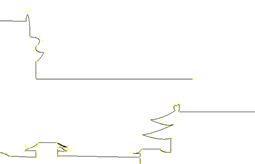这个图是照着个海报画的，水平一般尝试做画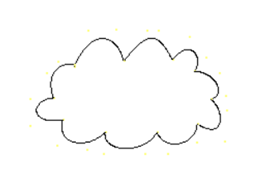周围会看到一些点。有颜色的话做图比较方便但美观性可能差一些

展开全文• 贝塞尔曲线matlab代码贝塞尔曲线 Matlab代码，用于生成2D贝塞尔曲线。 包含的m文件是用于计算De-Casteljau-Algorithm方面Bézier曲线的简单实现。 只要您引用作者，就可以在项目中随意使用基础代码。
• 上一讲讲解了伯恩斯坦多项式，现在就开始对Bezier曲线进行研究。首先Bezier曲线采用伯恩斯坦多项式作为基函数。 首先，我们定义Bezier曲线的表达式: C(t)=∑k=0nPkBkn(t)(1) \begin{aligned} \mathcal{C}(t) = \sum...

上一讲讲解了伯恩斯坦多项式，现在就开始对Bezier曲线进行研究。Bezier曲线采用伯恩斯坦多项式作为基函数。

首先，我们定义Bezier曲线的表达式:
C ( t ) = ∑ k = 0 n P k B k n ( t ) (1) \begin{aligned} \mathcal{C}(t) = \sum_{k=0}^n \mathbf{P}_k B_k^n(t) \end{aligned} \tag{1}

其中， ( P ) 0 ≤ k ≤ n \left( \mathbf{P} \right)_{0 \le k \le n} 是Bezier的控制顶点。虽然该表达式看起来很简单，但还是不那么直观，下面我们使用代码实现一下Bezier曲线。

### 计算伯恩斯坦多项式的值

要绘制出Bezier曲线，首先需要先求出 n n 次伯恩斯坦多项式在 t t 处的值，这里我们使用伯恩斯坦多项式的递归定义
B k , n ( t ) = ( 1 − t ) B k , n − 1 ( t ) + t B k − 1 , n − 1 ( t ) B_{k,n}(t)=(1-t)B_{k,n-1}(t)+tB_{k-1,n-1}(t)
代码实现如下

def all_bernstein(n, t):
b = np.zeros(n+1)
b = 1.
t1 = 1.-t
for j in range(1, n+1):
saved = 0.
for i in range(0, j):
tmp = b[i]
b[i] = saved + t1*tmp
saved = t*tmp
b[j] = saved
return b


### 计算Bezier曲线的值

我们根据式(1)，可以直接求得Bezier曲线在某一点处的值。伯恩斯坦多项式的次数根据控制顶点 P \mathbf{P} 的个数确定。

def point_on_bezier_curve(P,t):
n = len(P) - 1
b = all_bernstein(n, t)
c = 0.
for k in range(0, n+1):
c += b[k]*P[k]
return c


### 一些例子

下面例子中的ts表示在参数域的采样点

def example_1():
nt = 200
ts = np.linspace(0., 1., nt)

P = np.zeros((2, 2))
P[:, 0] = [0., 1.]
P[:, 1] = [1., 0.]

Q = np.zeros((nt, 2))
for i,t in enumerate(ts):
Q[i,:] = point_on_bezier_curve(P,t)

plt.plot(Q[:,0], Q[:,1], '-b')
plt.plot(P[:,0], P[:,1], '--ok', linewidth=0.7)

for i in range(0, 2):
x,y = P[i,:]
plt.text(x+0.05,y+0.05,'$\mathbf{P}_{' + str(i) + '}$')

plt.axis([-0.2, 1.2, -0.2, 1.2])

#ax = plt.axes()
#ax.set_aspect('equal', 'box')

example_1()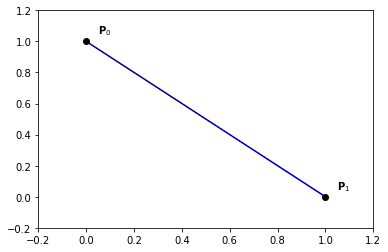def example_3a():
nt = 200
ts = np.linspace(0., 1., nt)

P = np.zeros((4, 2))
P[:, 0] = [1., 1., 0., -1.]
P[:, 1] = [0., 1., 1.,  0.]

Q = np.zeros((nt, 2))
for i,t in enumerate(ts):
Q[i,:] = point_on_bezier_curve(P,t)

plt.plot(Q[:,0], Q[:,1], '-b')
plt.plot(P[:,0], P[:,1], '--ok', linewidth=0.7)

for i in range(0, 3):
x,y = P[i,:]
plt.text(x+0.05,y+0.05,'$\mathbf{P}_{' + str(i) + '}$')
i = 3
x,y = P[i,:]
plt.text(x-0.05,y+0.05,'$\mathbf{P}_{' + str(i) + '}$')

plt.axis([-1.2, 1.2, -0.2, 1.2])

#ax = plt.axes()
#ax.set_aspect('equal', 'box')

example_3a()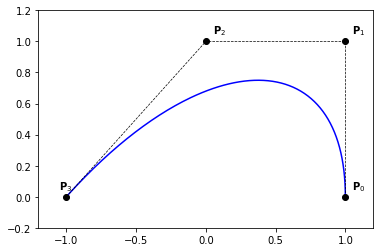#### 复合Bezier曲线

def plot_composite_bezier_curve():
n = 2

nt = 200
ts = np.linspace(0., 1., nt)

# ...
P = np.zeros((3, 2))
P[:, 0] = [1., 1., 0.]
P[:, 1] = [0., 1., 1.]

Q = np.zeros((nt, 2))
for i,t in enumerate(ts):
Q[i,:] = point_on_bezier_curve(P,t)

plt.plot(Q[:,0], Q[:,1], '-r')
plt.plot(P[:,0], P[:,1], '--or', linewidth=0.7)
# ...

# ...
P = np.zeros((3, 2))
P[:, 0] = [0., -1., 0.]
P[:, 1] = [1., -1., -1.]

Q = np.zeros((nt, 2))
for i,t in enumerate(ts):
Q[i,:] = point_on_bezier_curve(P,t)

plt.plot(Q[:,0], Q[:,1], '-b')
plt.plot(P[:,0], P[:,1], '--ob', linewidth=0.7)
# ...

# ...
P = np.zeros((3, 2))
P[:, 0] = [1., 1., 0.]
P[:, 1] = [0., -1., -1.]

Q = np.zeros((nt, 2))
for i,t in enumerate(ts):
Q[i,:] = point_on_bezier_curve(P,t)

plt.plot(Q[:,0], Q[:,1], '-g')
plt.plot(P[:,0], P[:,1], '--og', linewidth=0.7)
# ...

#plt.axis('equal')
#plt.title('Composite Bézier curve')

plot_composite_bezier_curve()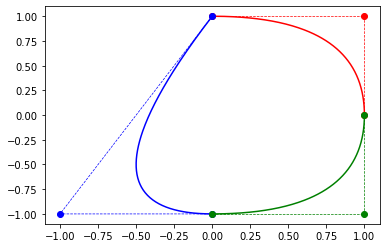### 一些Beizer曲线的性质

• 伯恩斯坦基函数次数=控制顶点数-1
• 控制顶点数=伯恩斯坦基函数的个数
• 伯恩斯坦基函数的个数=伯恩斯坦基函数次数+1
• 物理域的取值只和控制顶点的取值有关
展开全文• 绘制最小封闭圆和Beizer样条曲线 输出和详细信息在文件190201094-19020140-REPORT.docx中。 您可以在190201094-190201040.zip的mecproject文件夹中的ordinates.txt文件中输入点。 写下要点； 它必须是x1 x2 x3 x4 ...
• http://v.youku.com/v_show/id_XOTIyMzY1OTA0.htmlunity
• 《计算机图形学实验报告-实验3Bezier曲线》由会员分享，可在线阅读，更多相关《计算机图形学实验报告-实验3Bezier曲线(4页珍藏版)》请在人人文库网上搜索。1、计算机图形学实验报告班级 计算机工硕班 学号 姓名 王...
• [img=... 为何答案是 5 4 3 怎么判断的？ 求解释 ...还有就是知道起点，终点，控制点的坐标 用公式写beizer的方程时 P0 P 1 P2是要怎么代入....？x坐标和y坐标分别代入一次么
• ## 2次贝塞尔曲线算法

热门讨论 2011-10-25 17:50:09
2次贝塞尔曲线算法 用三次Bezier逼近圆弧:圆弧要等分成多少段 用三次Bezier逼近圆弧: 得到控制顶点数组 用三次Bezier逼近圆弧片段
• 应用python实现贝塞尔曲线，并应用wx python图像显示功能展现所画曲线。使用时需安装wx（内付安装程序）wxpython python程序
• 在绘制Hermite曲线的时候，发现如果令P0处的导数为3倍P1-P0，P3处的导数为3倍P3-P2，则P0,P1,P2,P3构成的Hermite曲线与P0,P1,P2,P3构成的Bezier曲线完全相同。 下面详细分析说明这一点 分析 通用的三次Hermite曲线的...Bezier OpenCV
• 贝塞尔曲线在CSS动画中和canvas、svg绘图中都是比较重要的一个东西！所以我来好好的小结一下关于它的一些东西。什么是贝塞尔曲线贝塞尔曲线于1962，由法国工程师皮埃尔·贝塞尔（Pierre Bézier）所广泛发表，他运用...
• 引言 这个问题在我做相关研究的时候一直很困惑，研究过很久很久，而且网上真的很难找到这方面的答案，在知乎问过（知乎那个问题就是我问的）...吴方法在曲线的参数形式与隐函数形式转换中的应用 这是知网的，在校...
• 本文给出 Hermite 与 Bezier 曲线互转的公式及其推导过程。 Bezier 曲线 p(t)=p0B0(t)+p1B1(t)+p2B2(t)+p3B3(t),t∈[0,1] \mathbf{p}(t) = \mathbf{p}_0 B_0(t) + \mathbf{p}_1 B_1(t) + \mathbf{p}_2 B_2(t) + \...
• 【XJTUSE计算机图形学】第三章 几何造型技术(2)——Bezier 曲线与曲面
• Bezier曲线是一款方便开发者做路径规划以及曲线运动的插件，支持可视化编辑、匀速曲线运动、支持各种缓动（InSine、OutSine等等）、支持多段曲线运动、绘制路径。方便开发者在项目组快速的实现曲线运动，不再为...typescript 匀速
• 计算机图形学 实验7 《复杂图形绘制-Bezier曲线与Hermite曲线》 一、实验目的 学习样条曲线的绘制。 二、实验内容 1、绘制Bezier曲线； 2、绘制Hermite曲线。 三、实验方法 Hermite曲线是给定曲线段的两个端点坐标...opengl
• 使用c# + GDI+进行SVG等绘图，绘制带控制点的Bezier曲线。可用于点阵图像及矢量图形（如SVG）绘图。GDI+ c# 算法
• MFC用B样条绘制分段Bezier曲线 将几何连续的分段多项式曲线统一采用B样条表示，是实现数据的统一管理。实现方式为将端节点重复度设置为K+1，内节点重复度设置为K，且控制点点个数减1的值为K的整数倍。 修改绘制代码...计算几何 C++
• ## 贝塞尔曲线简单介绍

万次阅读 多人点赞 2019-08-09 16:53:51
什么是贝塞尔曲线？ Bézier curve(贝塞尔曲线) 是应用于二维图形应用程序的数学曲线曲线定义：起始点、终止点（也称锚点）、控制点。通过调整控制点，贝塞尔曲线的形状会发生变化。 1962年，法国数学家Pierre B...规划控制
• 一、理论知识 ...自动驾驶中路径规划不仅要考虑一条安全（无碰撞）、高效（最短距离或 最短时间），还应该考虑自车动力学的约束，以及曲线的平滑。 贝塞尔曲线是一种常用的求解自动驾驶规划路径的方法。算法 动态规划 python 自动驾驶 人工智能
• ## 基于贝塞尔曲线的变道轨迹规划

千次阅读 多人点赞 2019-06-04 19:36:33
基于贝塞尔曲线的变道轨迹规划 车辆的换道与超车是驾驶员常见的驾驶操作之一，无人驾驶车辆在行驶过程中也会频繁的面临此工况，车辆行驶过程中必须根据行驶环境中车车之间的相对速度与距离，以及车辆周边其他环境的......